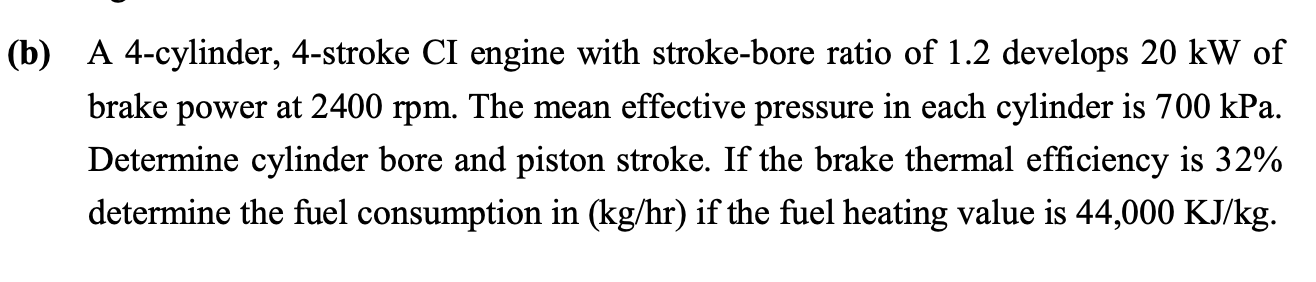# Question Solved1 Answer(b) A 4-cylinder, 4-stroke CI engine with stroke-bore ratio of 1.2 develops 20 kW of brake power at 2400 rpm. The mean effective pressure in each cylinder is 700 kPa. Determine cylinder bore and piston stroke. If the brake thermal efficiency is 32% determine the fuel consumption in (kg/hr) if the fuel heating value is 44,000 KJ/kg.6I5OSH The Asker · Mechanical EngineeringTranscribed Image Text: (b) A 4-cylinder, 4-stroke CI engine with stroke-bore ratio of 1.2 develops 20 kW of brake power at 2400 rpm. The mean effective pressure in each cylinder is 700 kPa. Determine cylinder bore and piston stroke. If the brake thermal efficiency is 32% determine the fuel consumption in (kg/hr) if the fuel heating value is 44,000 KJ/kg.
More
Transcribed Image Text: (b) A 4-cylinder, 4-stroke CI engine with stroke-bore ratio of 1.2 develops 20 kW of brake power at 2400 rpm. The mean effective pressure in each cylinder is 700 kPa. Determine cylinder bore and piston stroke. If the brake thermal efficiency is 32% determine the fuel consumption in (kg/hr) if the fuel heating value is 44,000 KJ/kg.